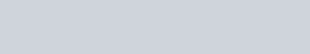respectively the sets of local minimum and local maximum points of the function,
Question:

If $S_{1}$ and $S_{2}$ are respectively the sets of local minimum and local maximum points of the function, $f(x)=9 x^{4}+12 x^{3}-36 x^{2}+25, x \in \mathrm{R}$, then :

1. (1) $\mathrm{S}_{1}=\{-2\} ; \mathrm{S}_{2}=\{0,1\}$

2. (2) $\mathrm{S}_{1}=\{-2,0\} ; \mathrm{S}_{2}=\{1\}$

3. (3) $\mathrm{S}_{1}=\{-2,1\} ; \mathrm{S}_{2}=\{0\}$

4. (4) $\mathrm{S}_{1}=\{-1\} ; \mathrm{S}_{2}=\{0,2\}$

Correct Option: , 3

Solution:

$f(x)=9 x^{4}+12 x^{3}-36 x^{2}+25$

$f^{\prime}(x)=36\left[x^{3}+x^{2}-2 x\right]=36 x(x-1)(x+2)$Here at $-2 \& 1, f^{\prime}(x)$ changes from negative value to positive value.

$\Rightarrow-2$ \& 1 are local minimum points. At $0, f^{\prime}(x)$ changes from positive value to negative value.

$\Rightarrow 0$ is the local maximum point.

Hence, $\mathrm{S}_{1}=\{-2,1\}$ and $\mathrm{S}_{2}=\{0\}$